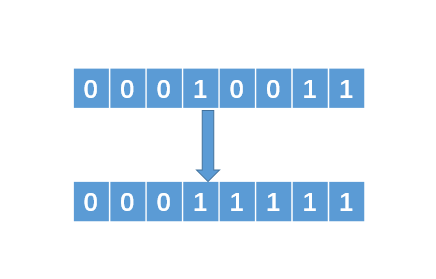# 【技巧总结】位运算装逼指南

### 判断奇偶数

if( n % 2) == 01
// n 是个奇数
}


if(n & 1 == 1){
// n 是个奇数。
}


### 2、交换两个数

int tmp = x;
x = y;
y = tmp;


x = x ^ y   // （1）
y = x ^ y   // （2）
x = x ^ y   // （3）


y = x^y = (xy)y = x(yy) = x^0 = x。 x 的值成功赋给了 y。

x = x^y = (xy)x = (xx)y = 0^y = y。

### 3、找出没有重复的数

123451234 = （11)(22)(33)(44)5= 00005 = 5。

int find(int[] arr){
int tmp = arr;
for(int i = 1;i < arr.length; i++){
tmp = tmp ^ arr[i];
}
return tmp;
}


### 4、m的n次方

int pow(int n){
int tmp = 1;
for(int i = 1; i <= n; i++) {
tmp = tmp * m;
}
return tmp;
}


m^1101 = m^0001 * m^0100 * m^1000。

int pow(int n){
int sum = 1;
int tmp = m;
while(n != 0){
if(n & 1 == 1){
sum *= tmp;
}
tmp *= tmp;
n = n >> 1;
}

return sum;
}


### 5、找出不大于N的最大的2的幂指数

int findN(int N){
int sum = 1;
while(true){
if(sum * 2 > N){
return sum;
}
sum = sum * 2;
}
}


1、找到最左边的 1，然后把它右边的所有 0 变成 12、把得到的数值加 1，可以得到 00100000即 00011111 + 1 = 00100000。

3、把 得到的 00100000 向右移动一位，即可得到 00010000，即 00100000 >> 1 = 00010000。

n |= n >> 1;
n |= n >> 2;
n |= n >> 4;


int findN(int n){
n |= n >> 1;
n |= n >> 2;
n |= n >> 4;
n |= n >> 8 // 整型一般是 32 位，上面我是假设 8 位。
return (n + 1) >> 1;
}


### 兄dei，如果觉得我写的不错，不妨帮个忙

1、关注我的原创微信公众号「帅地玩编程」，每天准时推送干货技术文章，专注于写算法 + 计算机基础知识（计算机网络+ 操作系统+数据库+Linux），听说关注了的不优秀也会变得优秀哦。

2、给俺点个赞呗，可以让更多的人看到这篇文章，顺便激励下我，嘻嘻。### 作者简洁10-062万+
06-01763
12-08783
11-103万+
08-281万+
01-241419
03-311470
10-161万+
05-161万+
04-05856
03-304987
11-261859
01-02100
04-034176
10-3163
12-213311
11-27111